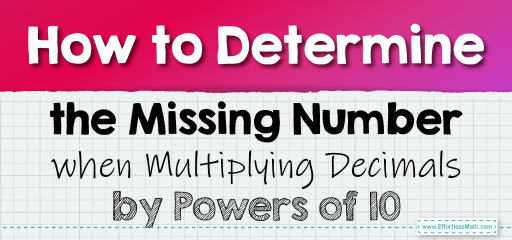# How to Determine the Missing Number when Multiplying Decimals by Powers of 10

Multiplying decimals by powers of 10 is a fundamental concept in mathematics. The beauty of powers of 10 is their ability to shift the decimal point, making multiplication straightforward. Let's explore how to determine the missing number when given a multiplication sentence involving decimals and powers of 10.Key Insight:

When multiplying by $$10$$, the decimal point moves one place to the right. For $$10^2$$ (or 100), it moves two places to the right, and so on.

## Multiplying by a Power of 10 with Decimals: Determine the Missing Number

### Example 1:

Complete the multiplication sentence: $$0.25 \times 10 = \_$$.

Solution Process:

Move the decimal point in $$0.25$$ one place to the right because we are multiplying by $$10$$.

$$0.25 \times 10 = 2.5$$.

The Absolute Best Book for 5th Grade Students

### Example 2:

Complete the multiplication sentence: $$3.45 \times 100 = \_$$.

Solution Process:

Move the decimal point in $$3.45$$ two places to the right because we are multiplying by $$10^2$$ (or 100).

$$3.45 \times 100 = 345$$.

Multiplying decimals by powers of 10 is a powerful tool that simplifies complex calculations. By understanding the relationship between decimals and powers of 10, you can quickly determine missing numbers in multiplication sentences. This skill not only enhances your mathematical prowess but also boosts your confidence in handling decimals. So, the next time you encounter a decimal multiplication problem involving powers of 10, remember the key insight and tackle it with ease!

### Practice Questions:

1. Determine the missing number: $$0.56 \times 10 = \_$$.

2. Complete the multiplication sentence: $$4.78 \times 1000 = \_$$.

3. Determine the missing number: $$0.009 \times 100 = \_$$.

4. Complete the multiplication sentence: $$5.67 \times 10^3 = \_$$.

5. Determine the missing number: $$0.123 \times 10^2 = \_$$.

A Perfect Book for Grade 5 Math Word Problems!

1. $$5.6$$

2. $$4780$$

3. $$0.9$$

4. $$5670$$

5. $$12.3$$

The Best Math Books for Elementary Students

### What people say about "How to Determine the Missing Number when Multiplying Decimals by Powers of 10 - Effortless Math: We Help Students Learn to LOVE Mathematics"?

No one replied yet.

X
30% OFF

Limited time only!

Save Over 30%

SAVE $5 It was$16.99 now it is \$11.99